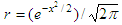# Normal Distribution

This algorithm (program in Matlab) calculates the probability and frequency of given values on a standard normal distribution curve (Gauss’ bell).

You have to enter the mean, the standard deviation and the value of interest. No special toolboxes or strange instructions are used.Standard Normal distribution

The shaded area represents the probability of ‘x’, and its frequency is ‘y’.
The normal probability is approximated using the following formula:

probability = 1 – r(a1t + a2t2 + a3t3) + eps

where:
a1 = 0.4361836
a2 = -0.1201676
a3 = 0.9372980t = 1/(1 + 0.33267x)

eps < 10-5

This is the Matlab code to accomplish the task,

% Clears screen and deletes all the variables in the workspace
clc; clear;

% Asks the user for input
m = input('Mean: ');
s = input(
'Std. Dev: ');
y = input(
'x = ');

% Calculates the frequency (y coordinate)
y = abs((y - m)/s);
r = exp(-y^2/2)/sqrt(2*pi);
str = [
'Frequency: ' num2str(r)];
disp(str)

z = y;
% Approximates probability (area under curve)
y = 1/(1 + 0.33267*abs(y));
a1 = 0.4361836;
a2 = -0.1201676;
a3 = 0.9372980;
t = 1 - r*(a1*y + a2*y^2 + a3*y^3);

if z < 0
t = 1 - t;

end

str = ['Probability: ' num2str(t)];
disp(str)

Example 1:

The mean instructions per millisecond (IPMS) of a certain type of modern computers is 150. The standard deviation is 15 IPMS. If the IPMS are normally distributed, what is the probability that a computer executes between 150 and 180 instructions per millisecond?

Easy. Run the Matlab code above and enter...

Mean: 150
Std. Dev: 15
x = 180

The handy Matlab response is

Frequency: 0.053991
Probability: 0.97724

Example 2:

What if we need to know the probability of an execution of 130 and 150 instructions per milliseconds for the same type of modern computers?

Don’t worry, Matlab is here...

Mean: 150
Std. Dev: 15
x = 130

Again, the handy response is

Frequency: 0.16401
Probability: 0.9088

From 'Normal Distribution to 'Probability and Statistics' Menu